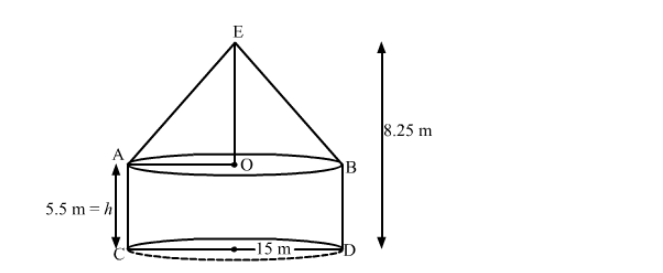# A military tent of height 8.25 m is in the form of a right circular cylinder of base diameter 30 m and height 5.5 m`
Question:

A military tent of height 8.25 m is in the form of a right circular cylinder of base diameter 30 m and height 5.5 m surmounted by a right circular cone of same base radius. Find the length of canvas used in making the tent, if the breadth of the canvas is 1.5 m.

Solution:We have,
the radii of bases of the cone and cylinder, r = 15 m,
the height of the cylinder, = 5.5 m,
the height of the tent = 8.25 m

Also, the height of the cone, $H=8.25-5.5=2.75 \mathrm{~m}$

The slant height of the cone, $l=\sqrt{r^{2}+H^{2}}$

$=\sqrt{15^{2}+2.75^{2}}$

$=\sqrt{225+7.5625}$

$=\sqrt{232.5625}$

$=15.25 \mathrm{~m}$

The area of the canvas used in making the tent $=$ CSA of cylinder $+$ CSA of cone

$=2 \pi r h+\pi r l$

$=\pi r(2 h+l)$

$=\frac{22}{7} \times 15(2 \times 5.5+15.25)$

$=\frac{22}{7} \times 15(11+15.25)$

$=\frac{22}{7} \times 15 \times 26.25$

$=1237.5 \mathrm{~m}^{2}$

As, the width of the canvas = 1.5 m

So, the length of the canvas $=\frac{237.5}{1.5}=825 \mathrm{~m}$

Hence, the length of the tent used for making the tent is 825 m.